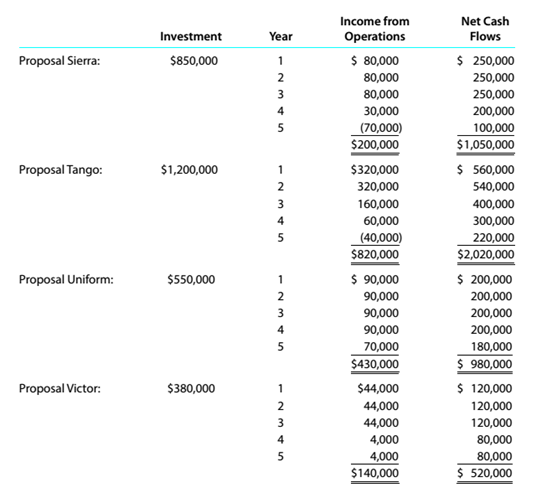Chapter 15, Problem 15.6.4P

Chapter
Section
Textbook Problem

Capital rationing decision involving four proposalsKopecky Industries Inc. is considering allocating a limited amount of capital investmentfunds among four proposals. The amount of proposed investment, estimated income fromoperations, and net cash flow for each proposal are as follows:The company’s capital rationing policy requires a maximum cash payback period of three years. In addition, a minimum average rate of return of 12% is required on all projects. 1f the preceding standards are met, the net present value method and present value indexes are used to rank the remaining proposals. InstructionsFor the proposals accepted for further analysis in part (3), compute the net present value. Use arate 0112% and the present value of $1 table appearing in thischapter. Round to the nearest dollar. To determine Concept Introduction: NPV: Net present value (NPV) is the method to evaluate the project feasibility. This method calculates the present value of cash inflows and outflows, and then calculates the net present value of the investment. A project should be accepted if it has a positive NPV. The formula to calculate the NPV is as follows: NPV = Present value of cash inflows Present value of cash out flows To Calculate: The Net Present Value for each accepted project Explanation The Net Present Value for each accepted project is calculated as follows:  Proposal Tango Net Cash Flows PVF (12%) PV A B =A*B Year 0$ (1,200,000) 1.0000 $(1,200,000) Year 1$ 560,000 0.8929 $500,000 Year 2$ 540,000 0.7972 $430,485 Year 3$ 400,000 0.7118 $284,712 Year 4$ 300,000 0.6355 $190,655 Year 5$ 220,000 0...

Still sussing out bartleby?

Check out a sample textbook solution.

See a sample solution

The Solution to Your Study Problems

Bartleby provides explanations to thousands of textbook problems written by our experts, many with advanced degrees!

Get Started